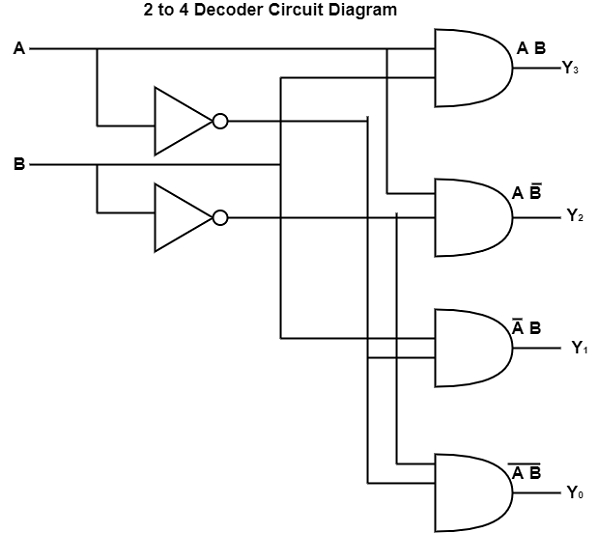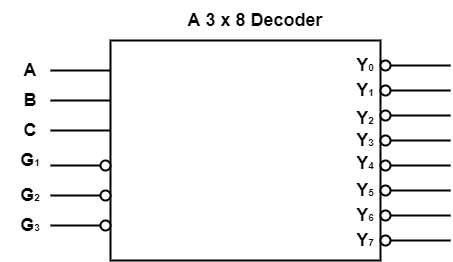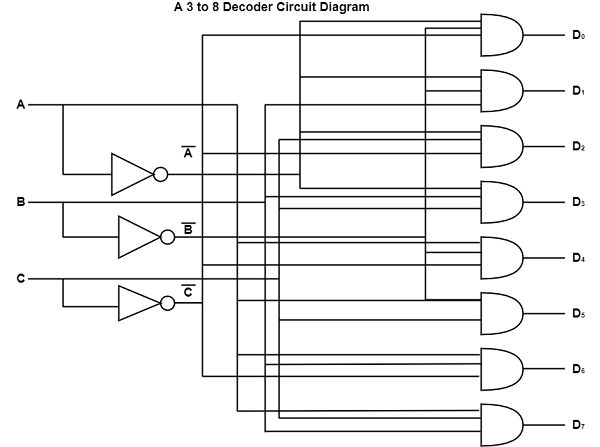# What is Binary Decoder?

A binary decoder is a decoder that has an n-bit binary input code and one activated output which is chosen from output codes. This decoder is accessible in instances where it is important to simulate directly one of the outputs based on an n-bit input value. In a 2 to 4 decoder, 2 inputs are decoded into four outputs, each output describing one of the min-terms of the 2 input variables. The two inverters support the complement of the inputs and each one of the four AND gates creates one of the min-terms.

The diagram shows the circuit diagram of a 2 to 4 Decoder.The truth table for a 2 x 4 Decoder which are as follows −

InputsOutputs
ENABY3Y2Y1Y0
0XX0000
1000001
1010010
1111000
1111000

## 3 to 8 Decoder

The 74x138 is a 3 to 8 decoder. It can receive three binary inputs, such as A, B, and C and it supports eight single active low outputs (Y0-Y7) when allowed. The device has three allowed inputs, such as two active low and one active high.

The diagram shows a 3 to 8 decoder.The diagram demonstrates the circuit diagram of a 3 to 8 decoder.The table shows the truth table of a 3 to 8 decoder.

ABCD0D1D2D3D4D5D6D7
00010000000
00101000000
01000100000
01100010000
10000001000
10100000100
11000000010
11100000001